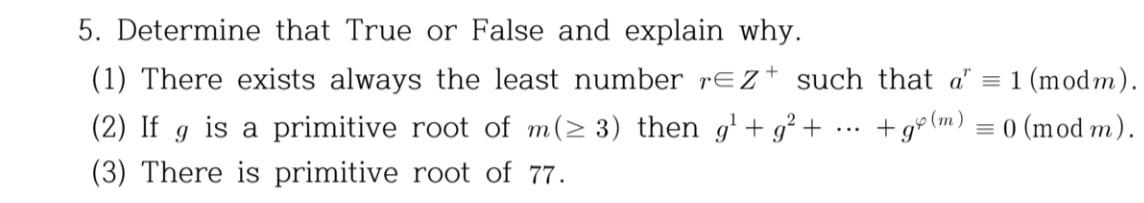Home / Expert Answers / Other Math / nbsp-5-determine-that-true-or-false-and-explain-why-1-there-exists-always-the-least-number-pa989

# (Solved):   5. Determine that True or False and explain why. (1) There exists always the least number ...5. Determine that True or False and explain why. (1) There exists always the least number $$r \in Z^{+}$$such that $$a^{r} \equiv 1(\bmod m)$$. (2) If $$g$$ is a primitive root of $$m(\geq 3)$$ then $$g^{1}+g^{2}+\cdots+g^{\varphi(m)} \equiv 0(\bmod m)$$. (3) There is primitive root of 77 .

We have an Answer from Expert